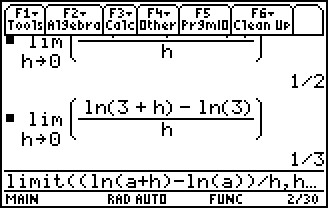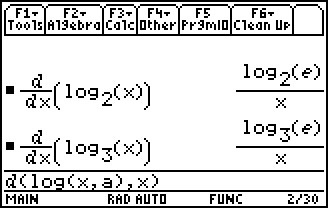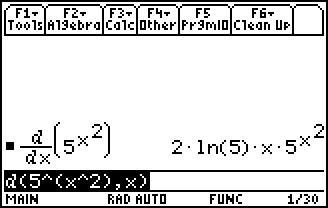# Activities

••• ##### Subject Area

• Math: Calculus: Derivatives

• ##### Author9-12

45 Minutes

• ##### Device
• TI-89 / TI-89 Titanium
• ##### Report an Issue

The Logarithmic Derivative#### Activity Overview

Students will determine the derivative of the function y = ln(x) and work with the derivative of both y = ln(u) and y = loga(u).

#### Key Steps

•Students begin the activity by finding the derivative of the natural log function at several values using the definition of a derivative. Students should begin seeing a pattern for the derivative of a natural logarithm function.

•Next, students will explore logarithmic functions that have a base other than e.

•Finally, students will use the Chain Rule to find the derivative of more complex exponential and logarithmic functions.